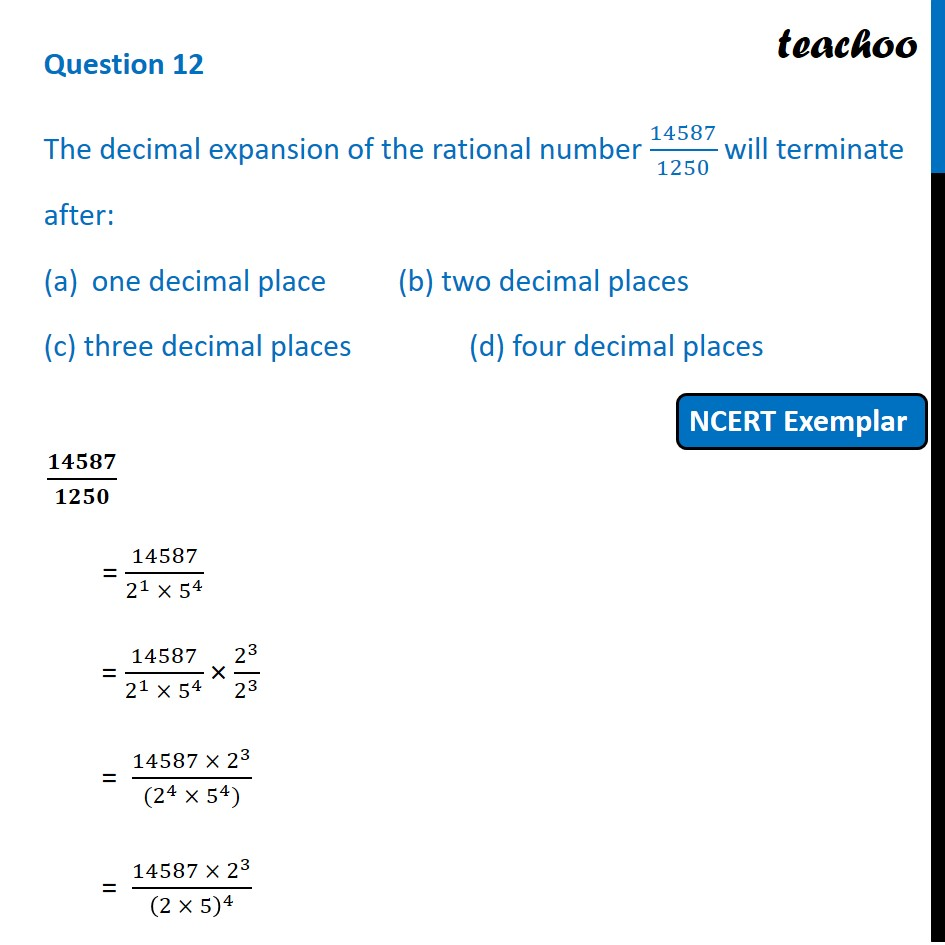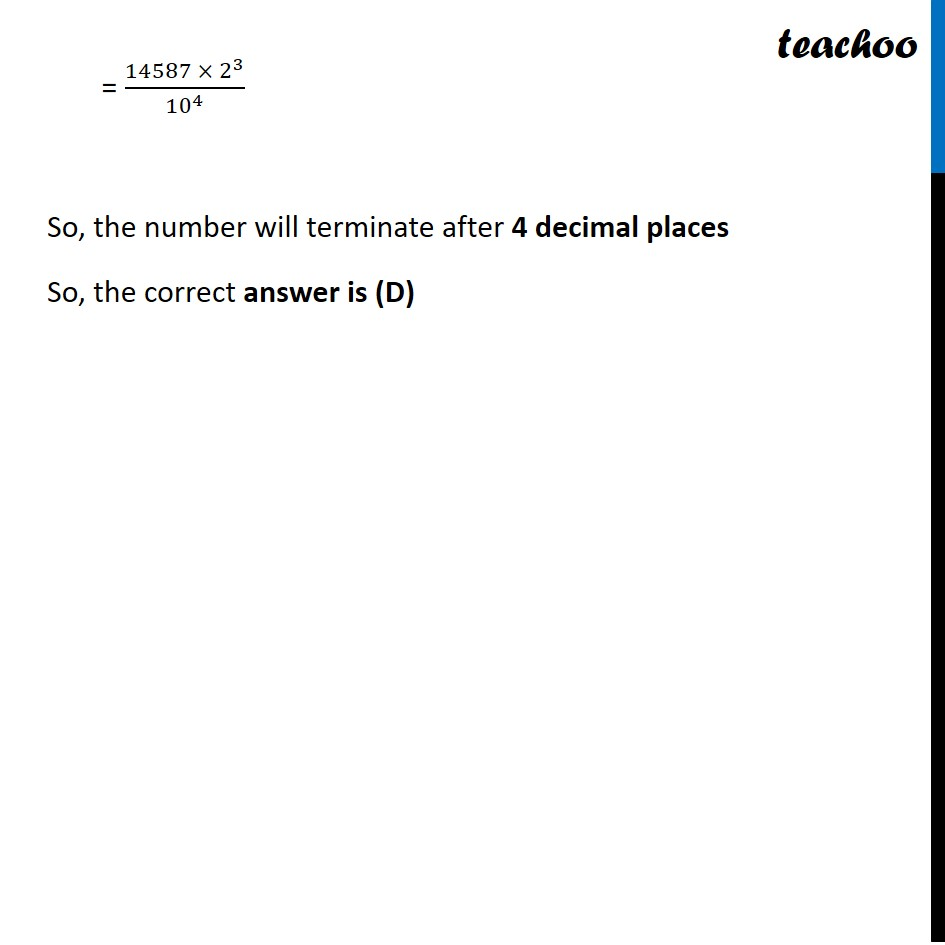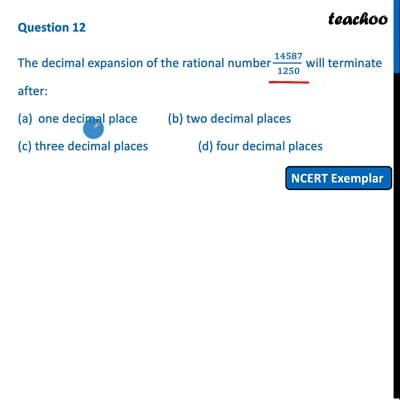MCQs from NCERT Exemplar

Chapter 1 Class 10 Real Numbers
Serial order wise

## (c) three decimal places  (d) four decimal placesThis video is only available for Teachoo black users

Learn in your speed, with individual attention - Teachoo Maths 1-on-1 Class

### Transcript

Question 12 The decimal expansion of the rational number 14587/1250 will terminate after: one decimal place (b) two decimal places (c) three decimal places (d) four decimal places 𝟏𝟒𝟓𝟖𝟕/𝟏𝟐𝟓𝟎 = 14587/(2^1 × 5^4 ) = 14587/(2^1 × 5^4 ) × 2^3/2^3 = (14587 × 2^3)/((2^4 × 5^4)) = (14587 × 2^3)/(2 × 5)^4 = (14587 × 2^3)/〖10〗^4 So, the number will terminate after 4 decimal places So, the correct answer is (D)